## Linear Algebra and Least Squares

### Linear Algebra Blocks

The Matrices and Linear Algebra library provides three large sublibraries containing blocks for linear algebra; Linear System Solvers, Matrix Factorizations, and Matrix Inverses. A fourth library, Matrix Operations, provides other essential blocks for working with matrices.

### Linear System Solvers

The Linear System Solvers library provides the following blocks for solving the system of linear equations AX = B:

Some of the blocks offer particular strengths for certain classes of problems. For example, the Cholesky Solver block is adapted for a square Hermitian positive definite matrix A, whereas the Backward Substitution block is suited for an upper triangular matrix A.

#### Solve AX=B Using the LU Solver Block

Open the `ex_lusolver_tut` model. The LU Solver block solves the equation Ax = b, whereand finds x to be the vector [-2 0 1]'.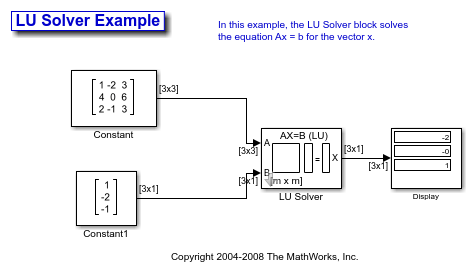You can verify the solution by using the Matrix Multiply block to perform the multiplication Ax, as shown in the following `ex_matrixmultiply_tut1` model.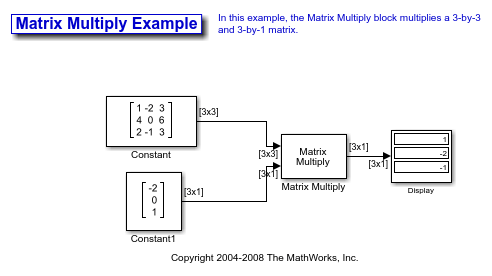### Matrix Factorizations

The Matrix Factorizations library provides the following blocks for factoring various kinds of matrices:

Some of the blocks offer particular strengths for certain classes of problems. For example, the Cholesky Factorization block is suited to factoring a Hermitian positive definite matrix into triangular components, whereas the QR Factorization is suited to factoring a rectangular matrix into unitary and upper triangular components.

#### Factor a Matrix into Upper and Lower Submatrices Using the LU Factorization Block

Open the `ex_lufactorization_tut` model. The LU Factorization block factors a matrix Ap into upper and lower triangular submatrices U and L, where Ap is row equivalent to input matrix A, where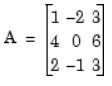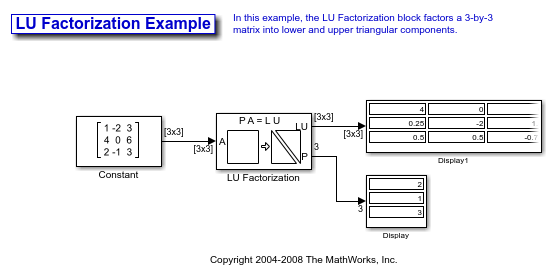The lower output of the LU Factorization P is the permutation index vector, which indicates that the factored matrix Ap is generated from A by interchanging the first and second rows.The upper output of the LU Factorization LU is a composite matrix containing the two submatrix factors U and L whose product LU is equal to Ap.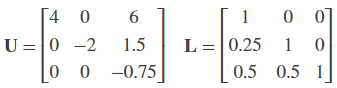You can check that LU = Ap with the Matrix Multiply block, as shown in the following `ex_matrixmultiply_tut2` model.### Matrix Inverses

The Matrix Inverses library provides the following blocks for inverting various kinds of matrices:

#### Find the Inverse of a Matrix Using the LU Inverse Block

Open the `ex_luinverse_tut` model. The LU Inverse block computes the inverse of input matrix A, whereand then forms the product inv(A)*A, which yields the identity matrix of order 3, as expected.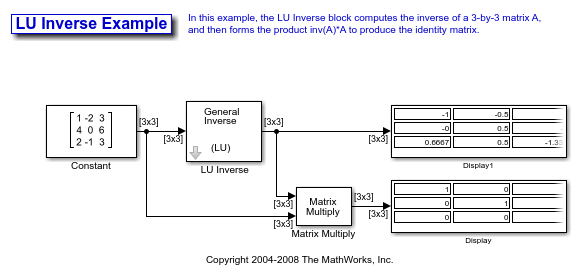As shown above, the computed inverse is